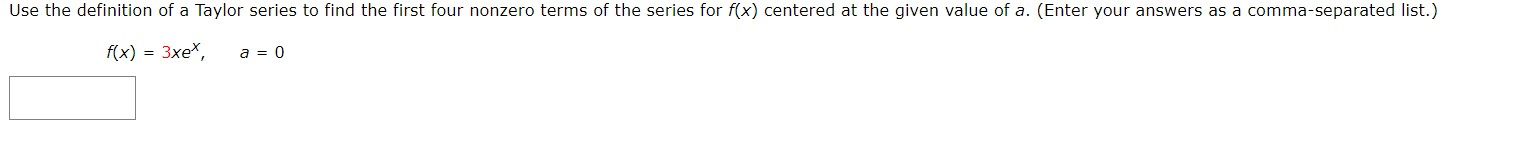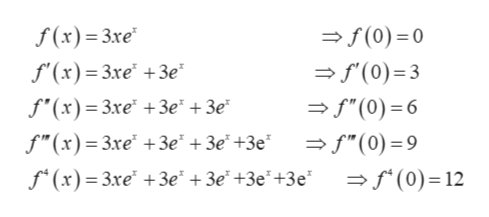# Use the definition of a Taylor series to find the first four nonzero terms of the series for f(x) centered at the given value of a. (Enter your answers as a comma-separated list.)f(x) 3xe*a 0

Question
88 viewshelp_outlineImage TranscriptioncloseUse the definition of a Taylor series to find the first four nonzero terms of the series for f(x) centered at the given value of a. (Enter your answers as a comma-separated list.) f(x) 3xe* a 0 fullscreen
check_circle

Step 1

A Taylor series is a series expansion of a function about a point. A Taylor series is an expansion of a real function  f(x) about a point x = a is given by

Step 2

Here in the given question a = 0:

Step 3

For the given function f(x) = 3xex...help_outlineImage Transcriptionclose>f(0)= 0 f(0) 3 f(x)= 3xe f'(x) 3xe 3e f'(x) 3xe +3e° +3e* f"(0) 6 f"(x)= 3xe +3e + 3e*+3e° - f"(0)= 9 f(x)= 3xe +3e +3e +3e*+3e f(0)= 12 fullscreen

### Want to see the full answer?

See Solution

#### Want to see this answer and more?

Solutions are written by subject experts who are available 24/7. Questions are typically answered within 1 hour.*

See Solution
*Response times may vary by subject and question.
Tagged in

### Math